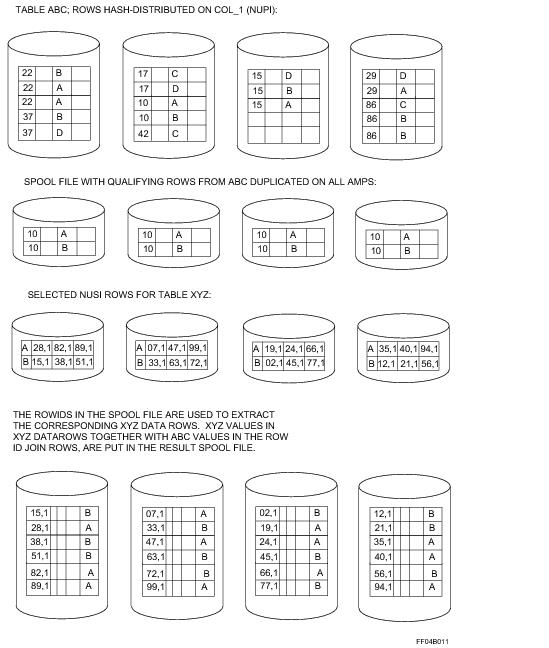# 15.10 - RowID Join - Teradata Database

## Teradata Database SQL Request and Transaction Processing

prodname
vrm_release
15.10
category
Programming Reference
User Guide
featnum
B035-1142-151K

The rowID join is a special form of the nested join. The Optimizer selects a rowID join instead of a nested join when the first condition in the query specifies a literal for the first table. This value is then used to select a small number of rows which are then equijoined with a secondary index from the second table.

The Optimizer can select a rowID join only if both of the following conditions are true:

• The WHERE clause condition must match another column of the first table to a NUSI, USI, or join index (if the join index has a rowID of the base table) of the second table.
• Only a subset of the NUSI or USI values from the second table are qualified via the join condition (this is referred to as a weakly selective index condition), and a nested join is done between the two tables to retrieve the rowIDs from the second table.
• Consider the following generic SQL query:

`     SELECT *`
`     FROM table_1, table_2`
`     WHERE table_1.NUPI = value`
`     AND   table_1.column = table_2.weakly_selective_NUSI;`

The process involved in solving the join steps for this request is as follows:

1 The qualifying table_1 rows are duplicated on all AMPS.

2 The value in the join column of a table_1 row is used to hash into the table_2 NUSI (similar to a nested join).

3 The rowIDs are extracted from the index subtable and placed into a spool together with the corresponding table_1 columns. This becomes the left table for the join.

4 When all table_1 rows have been processed, the spool is sorted into rowID sequence.

5 The rowIDs in the spool are then used to extract the corresponding table_2 data rows.

6 table_2 values in table_2 data rows are put in the results spool together with table_1 values in the rowID join rows.

Stages 2 and 3 are part of a nested join. Stages 4, 5, and 6 describe the rowID join.

The following graphic demonstrates a rowID join:Assume that you submit the following SELECT request. The first WHERE condition is on a NUPI and the second is on a NUSI. The Optimizer applies a rowID join to process the join.

`     SELECT *`
`     FROM table_1, table_2`
`     WHERE table_1.column_1 = 10`
`     AND   table_1.column_3 = table_2.column_5;`